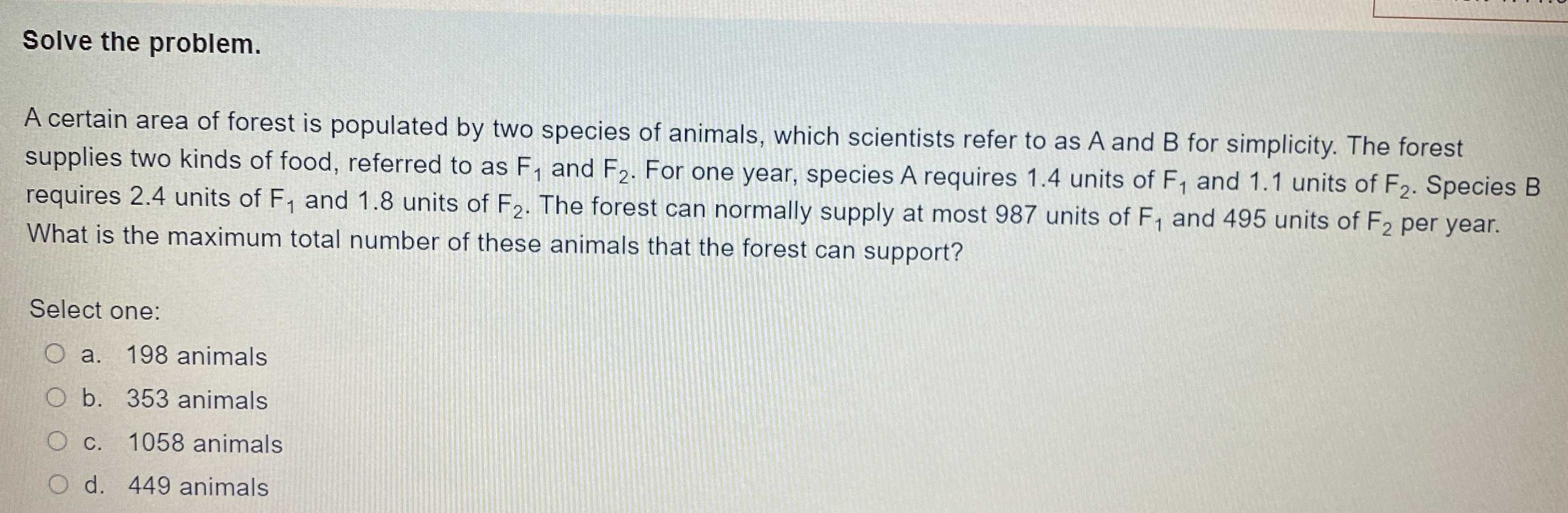### Still have math questions?

Algebra
QuestionSolve the problem. A certain area of forest is populated by two species of animals, which scientists refer to as $$A$$ and $$B$$ for simplicity. The forest supplies two kinds of food, referred to as $$F _ { 1 }$$ and $$F _ { 2 }$$ . For one year, species A requires $$1.4$$ units of $$F _ { 1 }$$ and $$1.1$$ units of $$F _ { 2 }$$ . Species $$B$$ requires $$2.4$$ units of $$F _ { 1 }$$ and $$1.8$$ units of $$F _ { 2 }$$ . The forest can normally supply at most $$987$$ units of $$F _ { 1 }$$ and $$495$$ units of $$F _ { 2 }$$ per year. What is the maximum total number of these animals that the forest can support?

Select one:

a. $$198$$ animals

b. $$353$$ animals

c. $$1058$$ animals

d. $$449$$ animals